# Python-Tkinter图形化界面设计（详细教程 ）

## 一.图形化界面设计的基本理解

Python自带了tkinter 模块，实质上是一种流行的面向对象的GUI工具包 TK 的Python编程接口，提供了快速便利地创建GUI应用程序的方法。其图像化编程的基本步骤通常包括：

○ 导入 tkinter 模块
○ 创建 GUI 根窗体
○ 添加人机交互控件并编写相应的函数。
○ 在主事件循环中等待用户触发事件响应。

## 2.1. 数据集导入

from tkinter import *
root= Tk()
root.title('我的第一个Python窗体')
root.geometry('240x240') # 这里的乘号不是 * ，而是小写英文字母 x
root.mainloop()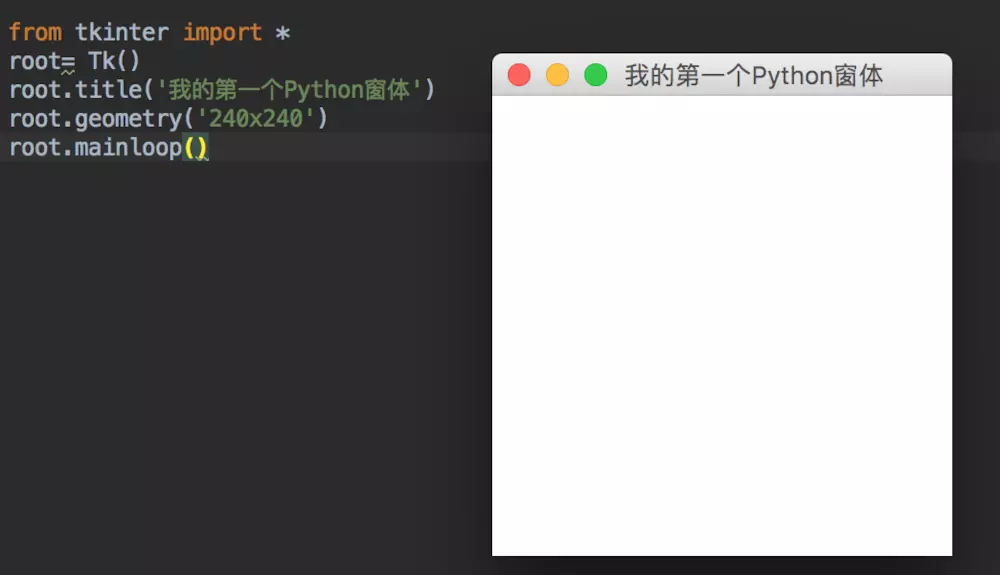## 2.2. tkinter 常用控件

#### 返回目录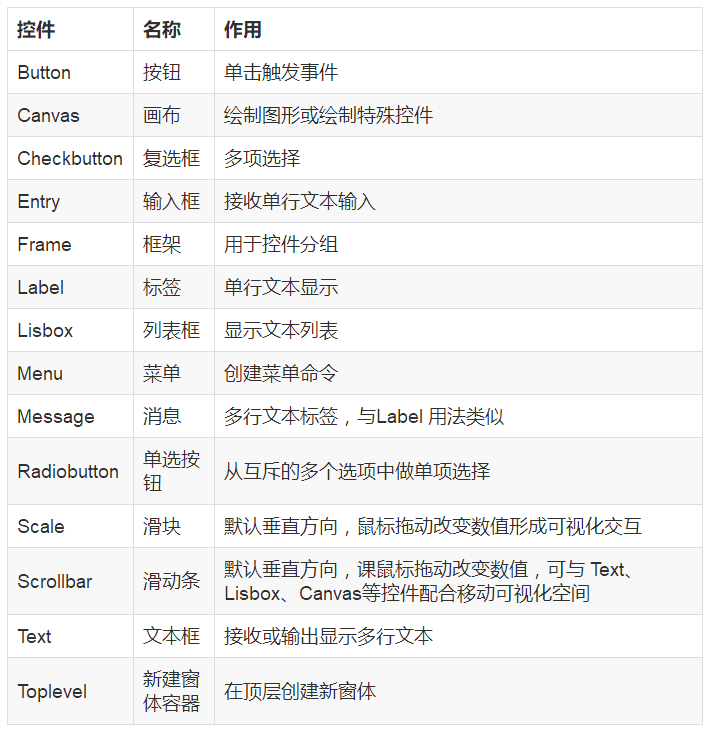## 2.2.1 控件的共同属性

#### 返回目录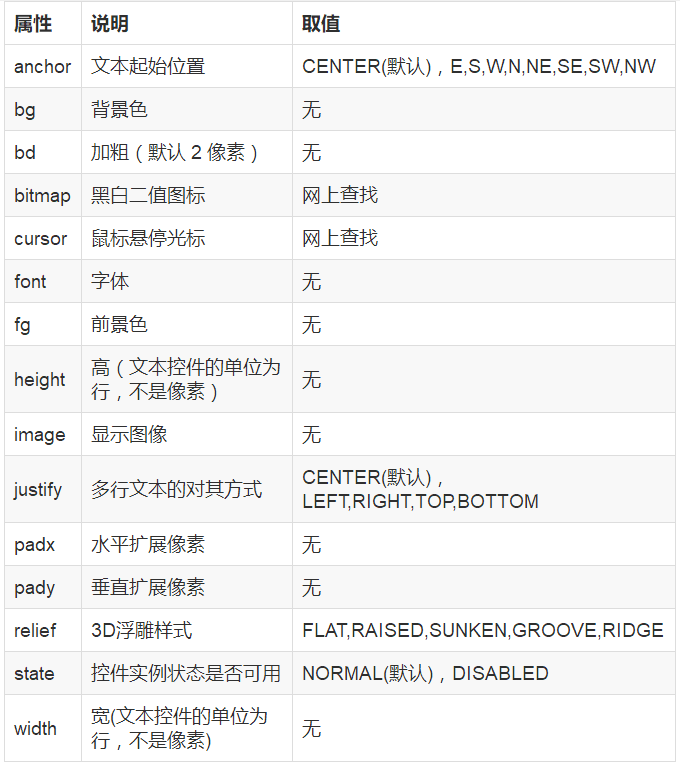from  tkinter import *
root = Tk()
lb = Label(root,text='我是第一个标签',\
bg='#d3fbfb',\
fg='red',\
font=('华文新魏',32),\
width=20,\
height=2,\
relief=SUNKEN)
lb.pack()
root.mainloop()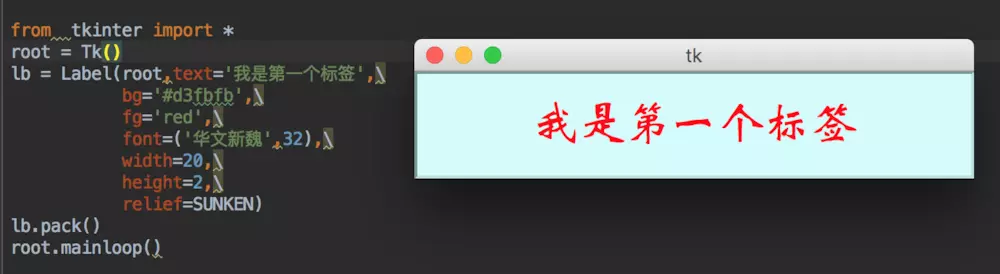Label(root,text='我是第一个标签',font='华文新魏').pack()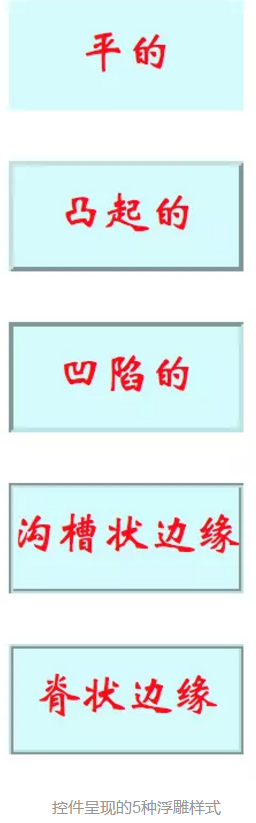## 2.3 控件布局

#### 返回目录

pack和grid请参考：https://www.jianshu.com/p/91844c5bca78

## 2.3.1 place()方法

#### 返回目录

x,y：控件实例在根窗体中水平和垂直方向上的其实位置（单位为像素）。注意，根窗体左上角为0,0,水平向右，垂直向下为正方向。
relx,rely：控件实例在根窗体中水平和垂直方向上起始布局的相对位置。即相对于根窗体宽和高的比例位置，取值在0.0~1.0之间。
height,width：控件实例本身的高度和宽度（单位为像素）。
relheight,relwidth：控件实例相对于根窗体的高度和宽度比例，取值在0.0~1.0之间。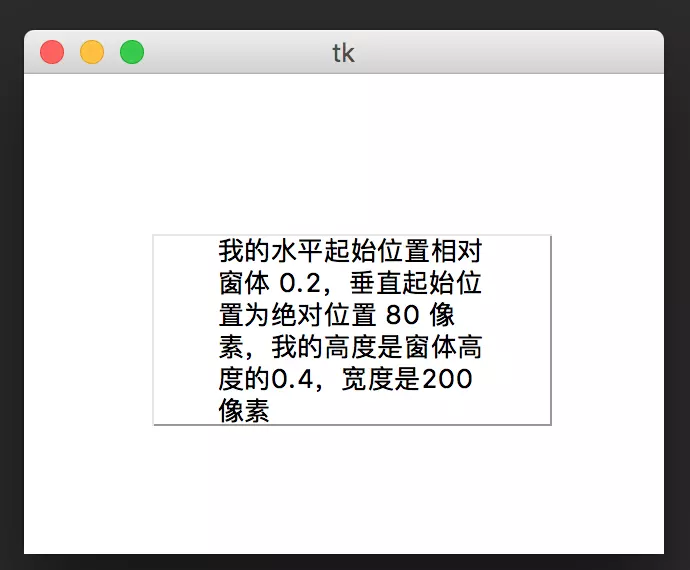from tkinter import *
root = Tk()
root.geometry('320x240')

msg1 = Message(root,text='''我的水平起始位置相对窗体 0.2，垂直起始位置为绝对位置 80 像素，我的高度是窗体高度的0.4，宽度是200像素''',relief=GROOVE)
msg1.place(relx=0.2,y=80,relheight=0.4,width=200)
root.mainloop()


## ○ 3.1.1 标签（Label）和 消息（Message）

#### 返回目录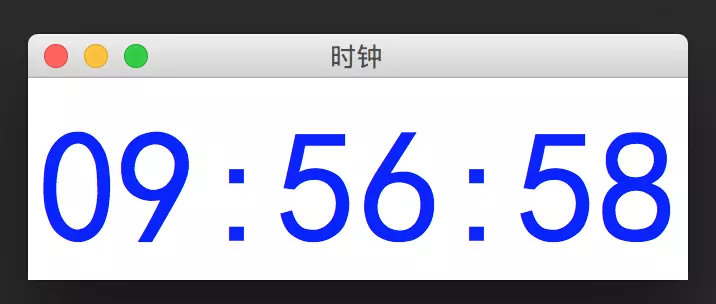import tkinter
import time

def gettime():
timestr = time.strftime("%H:%M:%S") # 获取当前的时间并转化为字符串
lb.configure(text=timestr)   # 重新设置标签文本
root.after(1000,gettime) # 每隔1s调用函数 gettime 自身获取时间

root = tkinter.Tk()
root.title('时钟')

lb = tkinter.Label(root,text='',fg='blue',font=("黑体",80))
lb.pack()
gettime()
root.mainloop()


import tkinter
import time

def gettime():
var.set(time.strftime("%H:%M:%S"))   # 获取当前时间
root.after(1000,gettime)   # 每隔1s调用函数 gettime 自身获取时间

root = tkinter.Tk()
root.title('时钟')
var=tkinter.StringVar()

lb = tkinter.Label(root,textvariable=var,fg='blue',font=("黑体",80))
lb.pack()
gettime()
root.mainloop()


## ○ 3.1.2 文本框（Text）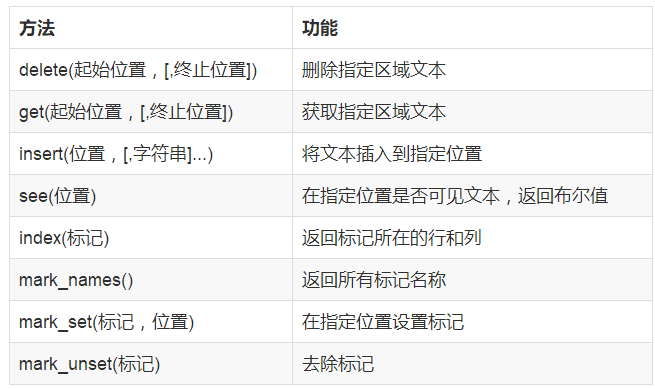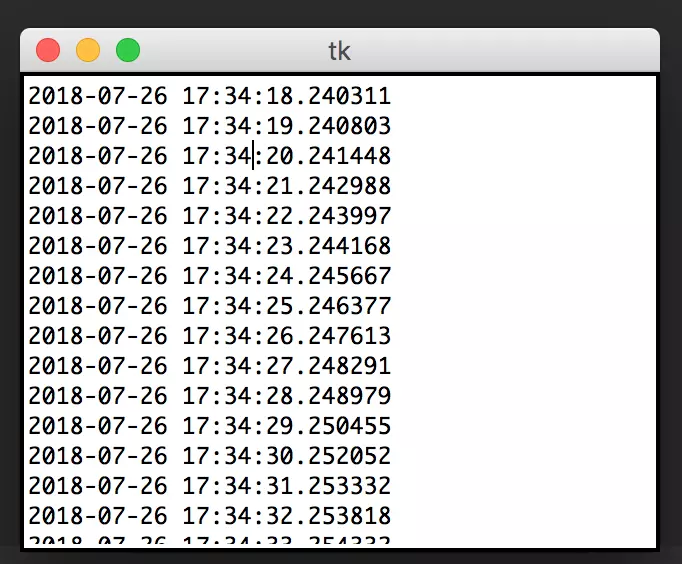from tkinter import *
import time
import datetime

def gettime():
s=str(datetime.datetime.now())+'\n'
txt.insert(END,s)
root.after(1000,gettime)  # 每隔1s调用函数 gettime 自身获取时间

root=Tk()
root.geometry('320x240')
txt=Text(root)
txt.pack()
gettime()
root.mainloop()


## 3.2 按钮(Button)

#### 返回目录

○ 直接调用函数。参数表达式为“command=函数名”，注意函数名后面不要加括号，也不能传递参数。如下面的command=run1：
○ 利用匿名函数调用函数和传递参数。参数的表达式为“command=lambda”:函数名（参数列表）。例如下面的："command=lambda:run2(inp1.get(),inp2.get())"。

○ 看下面的例子：1.从两个输入框去的输入文本后转为浮点数值进行加法运算，要求每次单击按钮产生的算是结果以文本的形式追加到文本框中，将原输入框清空。2.按钮方法一不传参数调用函数run1()实现，按钮“方法二”用lambda调用函数run2(x,y)同时传递参数实现。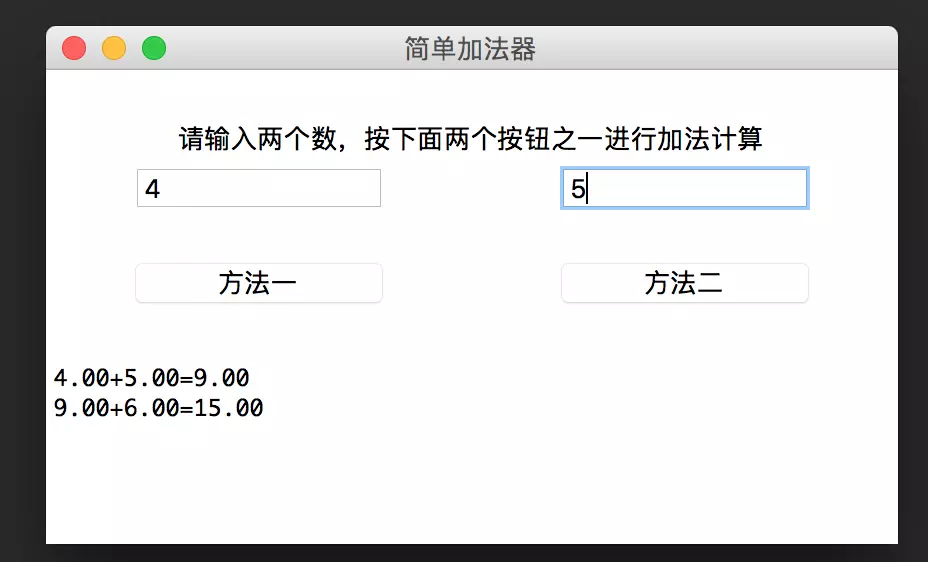from tkinter import *

def run1():
a = float(inp1.get())
b = float(inp2.get())
s = '%0.2f+%0.2f=%0.2f\n' % (a, b, a + b)
txt.insert(END, s)   # 追加显示运算结果
inp1.delete(0, END)  # 清空输入
inp2.delete(0, END)  # 清空输入

def run2(x, y):
a = float(x)
b = float(y)
s = '%0.2f+%0.2f=%0.2f\n' % (a, b, a + b)
txt.insert(END, s)   # 追加显示运算结果
inp1.delete(0, END)  # 清空输入
inp2.delete(0, END)  # 清空输入

root = Tk()
root.geometry('460x240')
root.title('简单加法器')

lb1 = Label(root, text='请输入两个数，按下面两个按钮之一进行加法计算')
lb1.place(relx=0.1, rely=0.1, relwidth=0.8, relheight=0.1)
inp1 = Entry(root)
inp1.place(relx=0.1, rely=0.2, relwidth=0.3, relheight=0.1)
inp2 = Entry(root)
inp2.place(relx=0.6, rely=0.2, relwidth=0.3, relheight=0.1)

# 方法-直接调用 run1()
btn1 = Button(root, text='方法一', command=run1)
btn1.place(relx=0.1, rely=0.4, relwidth=0.3, relheight=0.1)

# 方法二利用 lambda 传参数调用run2()
btn2 = Button(root, text='方法二', command=lambda: run2(inp1.get(), inp2.get()))
btn2.place(relx=0.6, rely=0.4, relwidth=0.3, relheight=0.1)

# 在窗体垂直自上而下位置60%处起，布局相对窗体高度40%高的文本框
txt = Text(root)
txt.place(rely=0.6, relheight=0.4)

root.mainloop()


## 3.3 单选按钮

#### 返回目录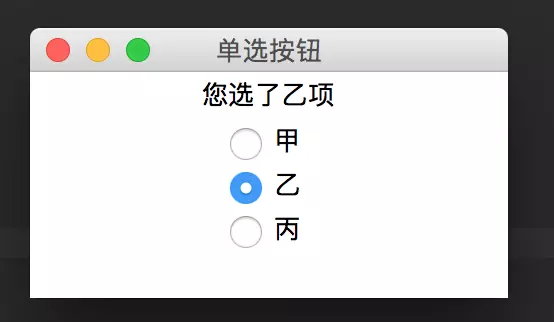from tkinter import *
def Mysel():
dic = {0:'甲',1:'乙',2:'丙'}
s = "您选了" + dic.get(var.get()) + "项"
lb.config(text = s)

root = Tk()
root.title('单选按钮')
lb = Label(root)
lb.pack()

var = IntVar()
rd1.pack()

rd2.pack()

rd3.pack()

root.mainloop()


## 3.4 复选框

#### 返回目录

(Checkbutton) 是为了返回多个选项值的交互控件，通常不直接触发函数的执行。该控件除具有共有属性外，还具有显示文本（text）、返回变量（variable）、选中返回值（onvalue）和未选中默认返回值（offvalue）等重要属性。返回变量variable=var 通常可以预先逐项分别声明变量的类型var=IntVar() (默认)或 var=StringVar(), 在所调用的函数中方可分别调用 var.get()方法 取得被选中实例的 onvalue或offvalue值。复选框实例通常还可分别利用 select()、deselect()和 toggle() 方法对其进行选中、清除选中和反选操作。

○ 如下的例子： 利用复选框实现，单击OK，可以将选中的结果显示在标签上。效果如下：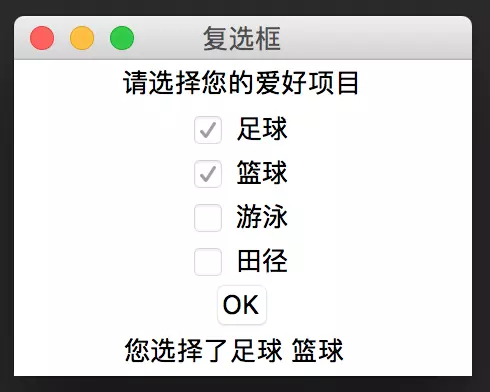○ 方法：利用函数中的 if-else 分支实现多项显示

from tkinter import *
import tkinter

def run():
if(CheckVar1.get()==0 and CheckVar2.get()==0 and CheckVar3.get()==0 and CheckVar4.get()==0):
s = '您还没选择任何爱好项目'
else:
s1 = "足球" if CheckVar1.get()==1 else ""
s2 = "篮球" if CheckVar2.get() == 1 else ""
s3 = "游泳" if CheckVar3.get() == 1 else ""
s4 = "田径" if CheckVar4.get() == 1 else ""
s = "您选择了%s %s %s %s" % (s1,s2,s3,s4)
lb2.config(text=s)

root = tkinter.Tk()
root.title('复选框')
lb1=Label(root,text='请选择您的爱好项目')
lb1.pack()

CheckVar1 = IntVar()
CheckVar2 = IntVar()
CheckVar3 = IntVar()
CheckVar4 = IntVar()

ch1 = Checkbutton(root,text='足球',variable = CheckVar1,onvalue=1,offvalue=0)
ch2 = Checkbutton(root,text='篮球',variable = CheckVar2,onvalue=1,offvalue=0)
ch3 = Checkbutton(root,text='游泳',variable = CheckVar3,onvalue=1,offvalue=0)
ch4 = Checkbutton(root,text='田径',variable = CheckVar4,onvalue=1,offvalue=0)

ch1.pack()
ch2.pack()
ch3.pack()
ch4.pack()

btn = Button(root,text="OK",command=run)
btn.pack()

lb2 = Label(root,text='')
lb2.pack()
root.mainloop()


## 3.5.1 列表框

#### 返回目录

(Listbox) 可供用户单选或多选所列条目以形成人机交互。列表框控件的主要方法见下面的表：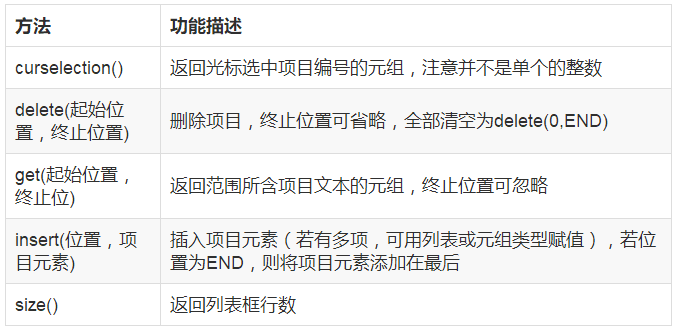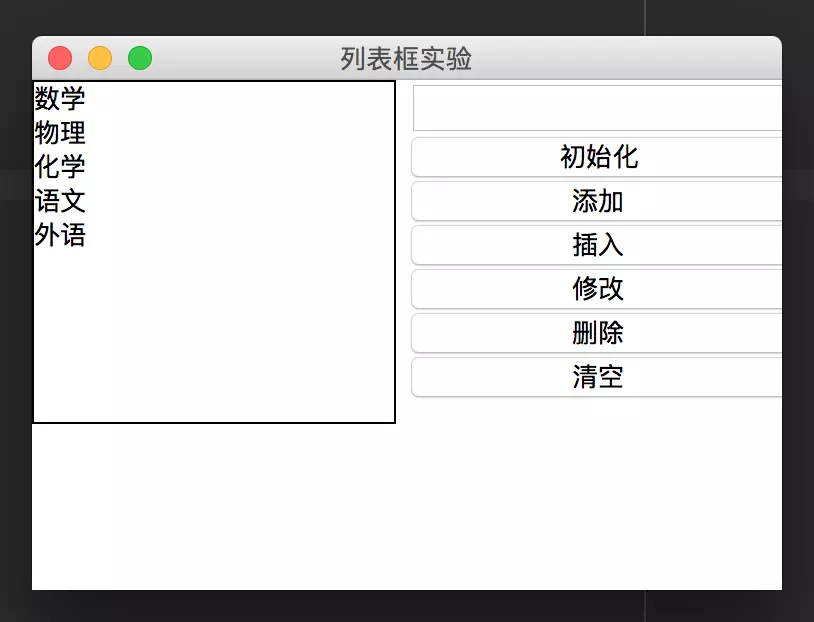from tkinter import *
def ini():
Lstbox1.delete(0,END)
list_items = ["数学","物理","化学","语文","外语"]
for item in list_items:
Lstbox1.insert(END,item)

def clear():
Lstbox1.delete(0,END)

def ins():
if entry.get() != '':
if Lstbox1.curselection() == ():
Lstbox1.insert(Lstbox1.size(),entry.get())
else:
Lstbox1.insert(Lstbox1.curselection(),entry.get())

def updt():
if entry.get() != '' and Lstbox1.curselection() != ():
selected=Lstbox1.curselection()
Lstbox1.delete(selected)
Lstbox1.insert(selected,entry.get())

def delt():
if Lstbox1.curselection() != ():
Lstbox1.delete(Lstbox1.curselection())

root = Tk()
root.title('列表框实验')
root.geometry('320x240')

frame1 = Frame(root,relief=RAISED)
frame1.place(relx=0.0)

frame2 = Frame(root,relief=GROOVE)
frame2.place(relx=0.5)

Lstbox1 = Listbox(frame1)
Lstbox1.pack()

entry = Entry(frame2)
entry.pack()

btn1 = Button(frame2,text='初始化',command=ini)
btn1.pack(fill=X)

btn2 = Button(frame2,text='添加',command=ins)
btn2.pack(fill=X)

btn3 = Button(frame2,text='插入',command=ins) # 添加和插入功能实质上是一样的
btn3.pack(fill=X)

btn4 = Button(frame2,text='修改',command=updt)
btn4.pack(fill=X)

btn5 = Button(frame2,text='删除',command=delt)
btn5.pack(fill=X)

btn6 = Button(frame2,text='清空',command=clear)
btn6.pack(fill=X)

root.mainloop()


## 3.5.2 组合框

#### 返回目录

(Combobox) 实质上是带文本框的上拉列表框，其功能也将是Python 的列表类型数据可视化呈现，并提供用户单选或多选所列条目以形成人机交互。在图形化界面设计时，由于其具有灵活的界面，因此往往比列表框更受喜爱。但该控件并不包含在 tkinter 模块中，而是与 TreeView、Progressbar、Separator等控件一同包含在tkinter 的子模块ttk中。如果使用该控件，应先与from tkinter import ttk 语句引用ttk子模块，然后创建组合框实例： 实例名=Combobox(根对象,[属性列表])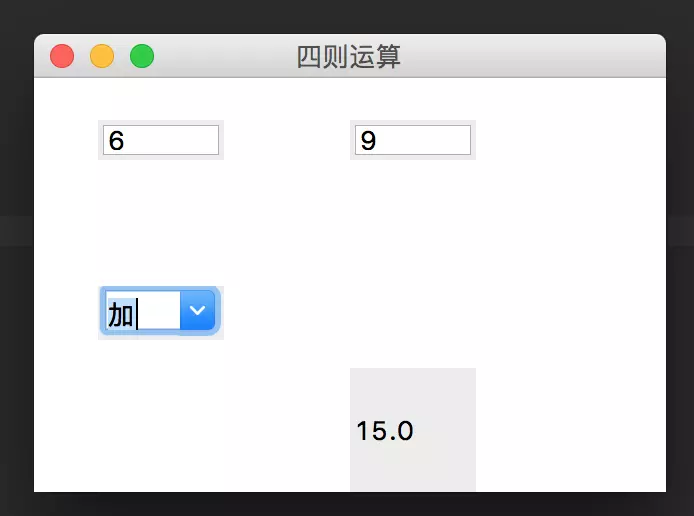from tkinter.ttk import *

def calc(event):
a = float(t1.get())
b = float(t2.get())
dic = {0:a+b,1:a-b,2:a*b,3:a/b}
c = dic[comb.current()]
lbl.config(text=str(c))

root = Tk()
root.title('四则运算')
root.geometry('320x240')

t1 = Entry(root)
t1.place(relx=0.1,rely=0.1,relwidth=0.2,relheight=0.1)

t2 = Entry(root)
t2.place(relx=0.5,rely=0.1,relwidth=0.2,relheight=0.1)

var = StringVar()

comb = Combobox(root,textvariable=var,values=['加','减','乘','除',])
comb.place(relx=0.1,rely=0.5,relwidth=0.2)
comb.bind('<<ComboboxSelected>>',calc)

lbl=Label(root,text='结果')
lbl.place(relx=0.5,rely=0.7,relwidth=0.2,relheight=0.3)

root.mainloop()


## 3.6 滑块

#### 返回目录

(Scale) 是一种 直观地进行数值输入的交互控件，其主要属性见下表：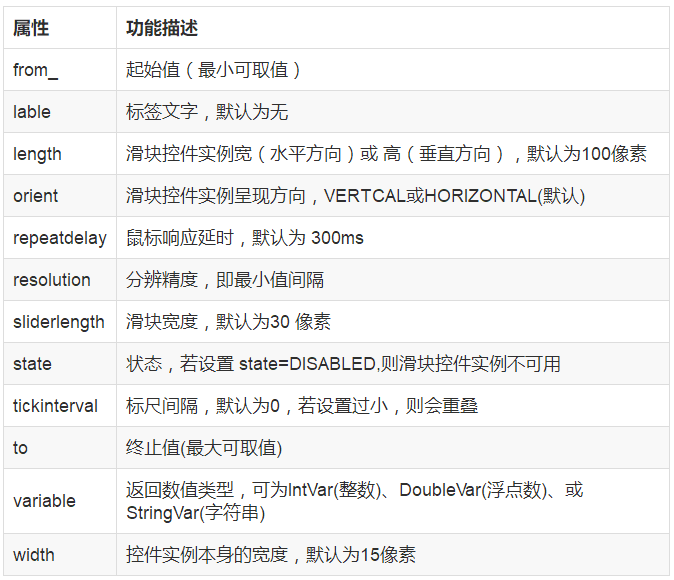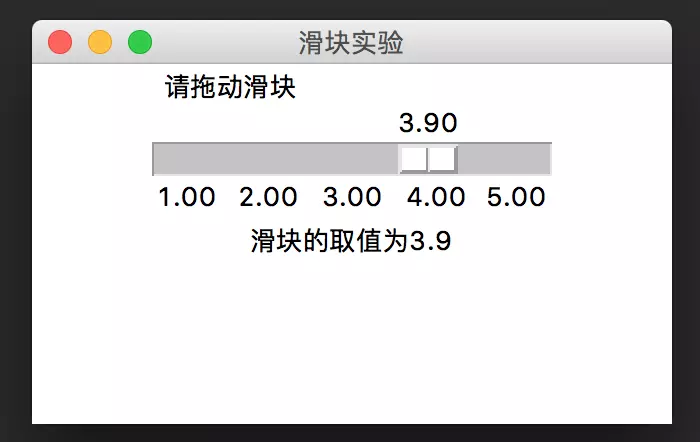from tkinter  import  *

def show(event):
s = '滑块的取值为' + str(var.get())
lb.config(text=s)

root = Tk()
root.title('滑块实验')
root.geometry('320x180')
var=DoubleVar()
scl = Scale(root,orient=HORIZONTAL,length=200,from_=1.0,to=5.0,label='请拖动滑块',tickinterval=1,resolution=0.05,variable=var)
scl.bind('<ButtonRelease-1>',show)
scl.pack()

lb = Label(root,text='')
lb.pack()

root.mainloop()


## 3.7 菜单

#### 返回目录

菜单实例名=Menu(根窗体)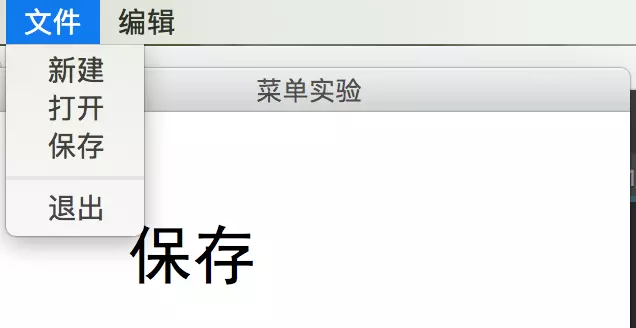from tkinter import *

def new():
s = '新建'
lb1.config(text=s)

def ope():
s = '打开'
lb1.config(text=s)

def sav():
s = '保存'
lb1.config(text=s)

def cut():
s = '剪切'
lb1.config(text=s)

def cop():
s = '复制'
lb1.config(text=s)

def pas():
s = '粘贴'
lb1.config(text=s)

root = Tk()
root.title('菜单实验')
root.geometry('320x240')

lb1 = Label(root,text='显示信息',font=('黑体',32,'bold'))
lb1.place(relx=0.2,rely=0.2)

root.mainloop()


## 3.8 子窗体

#### 返回目录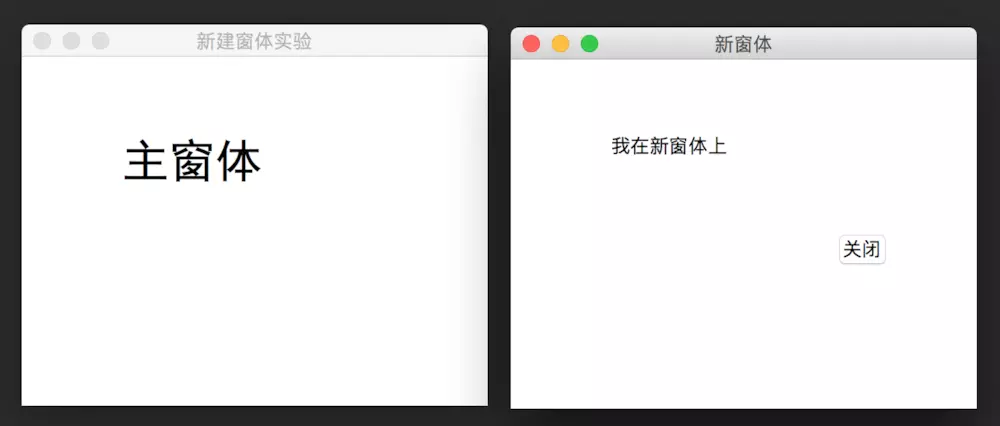from tkinter import *

def newwind():
winNew = Toplevel(root)
winNew.geometry('320x240')
winNew.title('新窗体')
lb2 = Label(winNew,text='我在新窗体上')
lb2.place(relx=0.2,rely=0.2)
btClose=Button(winNew,text='关闭',command=winNew.destroy)
btClose.place(relx=0.7,rely=0.5)

root = Tk()
root.title('新建窗体实验')
root.geometry('320x240')

lb1 = Label(root,text='主窗体',font=('黑体',32,'bold'))
lb1.place(relx=0.2,rely=0.2)

root.mainloop()


## 3.9.1 交互对话框

#### 返回目录

(一)、消息对话框: 引用 tkinter.messagebox 包，可使用消息对话框函数。执行这些函数，可弹出模式消息对话框，并根据用户的响应但会一个布尔值。其通式为：

消息对话框函数(<title=标题文本>,<message=消息文本>,[其他参数])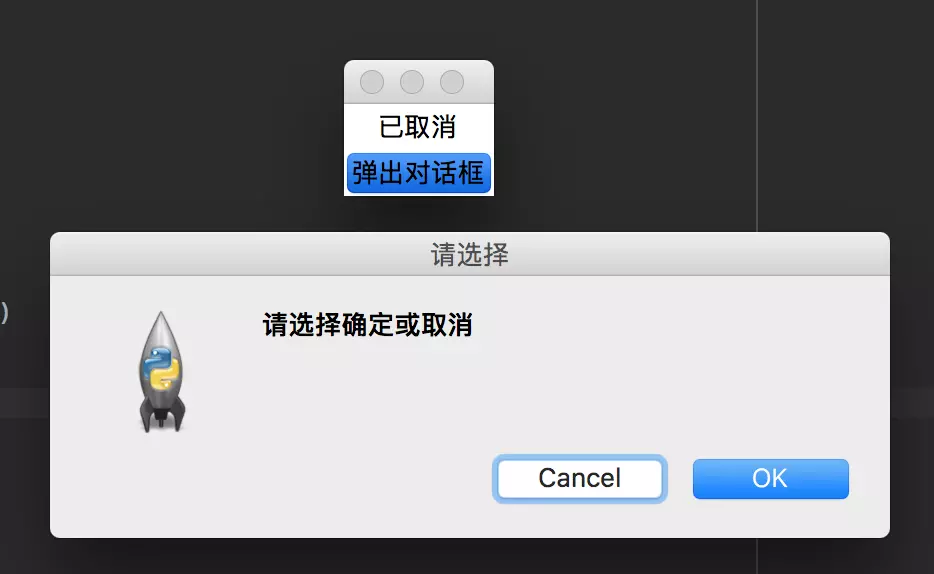from tkinter import *
import tkinter.messagebox

def xz():
lb.config(text='已确认')
else:
lb.config(text='已取消')

root = Tk()

lb = Label(root,text='')
lb.pack()
btn=Button(root,text='弹出对话框',command=xz)
btn.pack()
root.mainloop()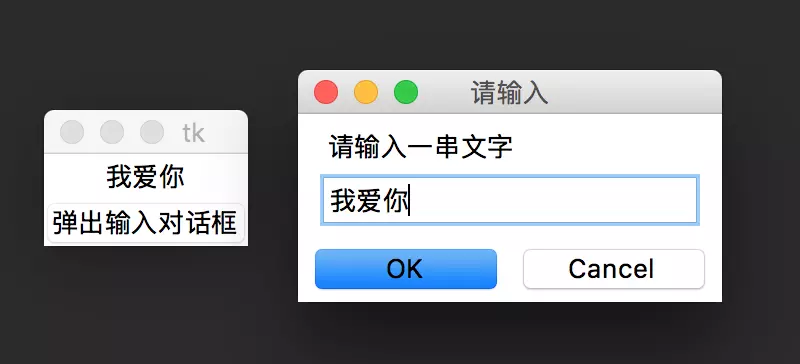from tkinter.simpledialog import *

def xz():
lb.config(text=s)

root = Tk()

lb = Label(root,text='')
lb.pack()
btn=Button(root,text='弹出输入对话框',command=xz)
btn.pack()
root.mainloop()


## 3.9.2 文件选择对话框

#### 返回目录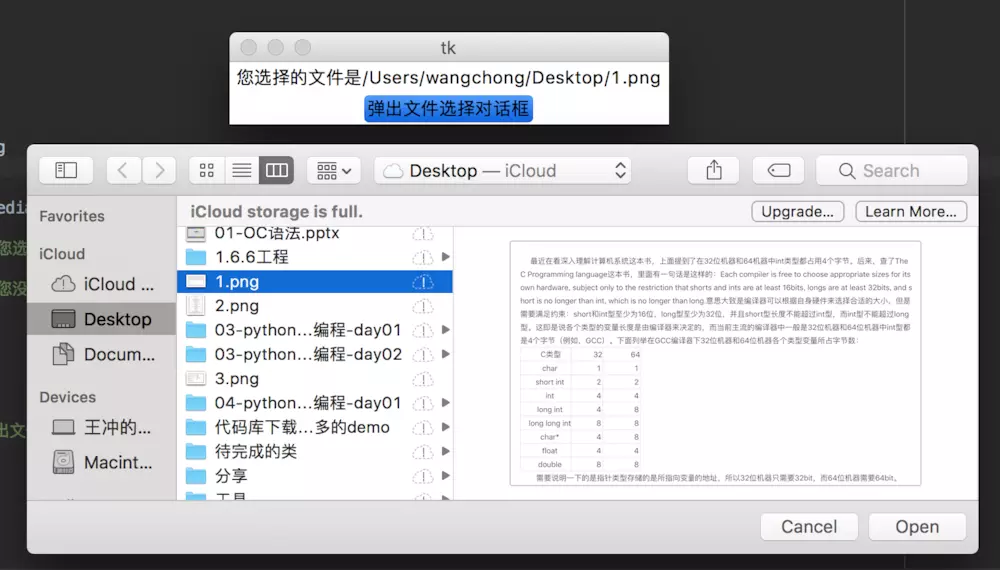from tkinter import *
import tkinter.filedialog

def xz():
if filename != '':
lb.config(text='您选择的文件是'+filename)
else:
lb.config(text='您没有选择任何文件')

root = Tk()

lb = Label(root,text='')
lb.pack()
btn=Button(root,text='弹出文件选择对话框',command=xz)
btn.pack()
root.mainloop()


## 3.9.3、颜色选择对话框

#### 返回目录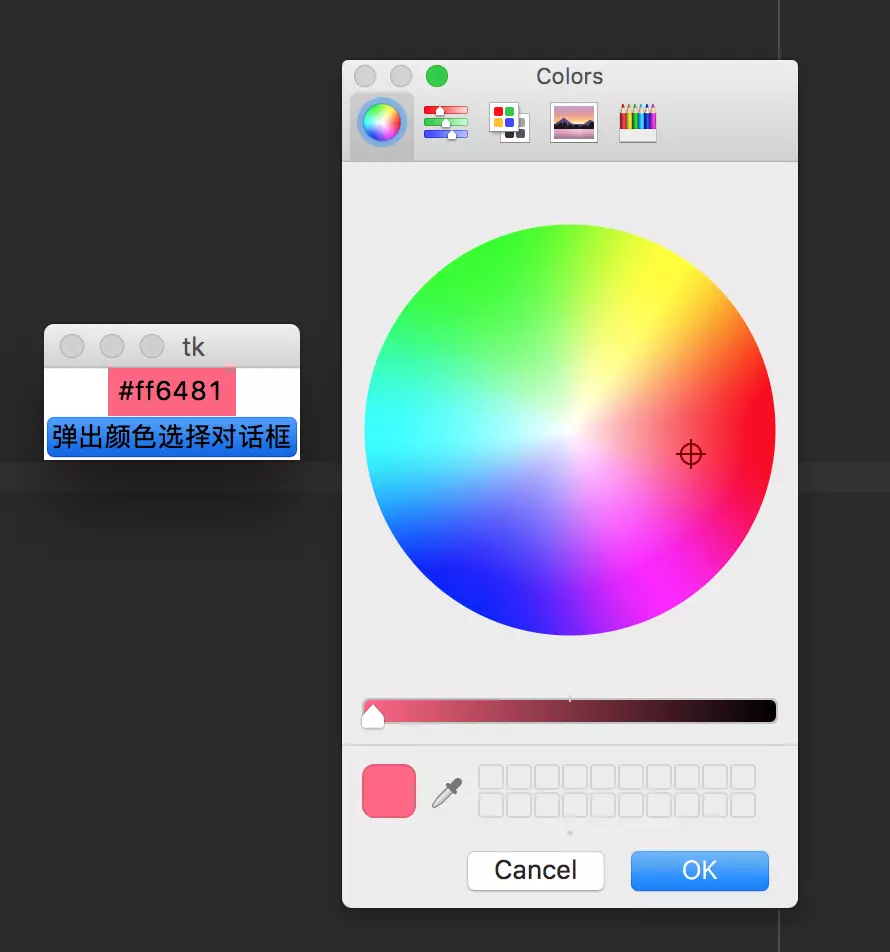from tkinter import *
import tkinter.colorchooser

def xz():
colorstr=str(color)
print('打印字符串%s 切掉后=%s' % (colorstr,colorstr[-9:-2]))
lb.config(text=colorstr[-9:-2],background=colorstr[-9:-2])

root = Tk()

lb = Label(root,text='请关注颜色的变化')
lb.pack()
btn=Button(root,text='弹出颜色选择对话框',command=xz)
btn.pack()
root.mainloop()


## 四、事件响应

#### 返回目录

控件实例.bind(<事件代码>,<函数名>)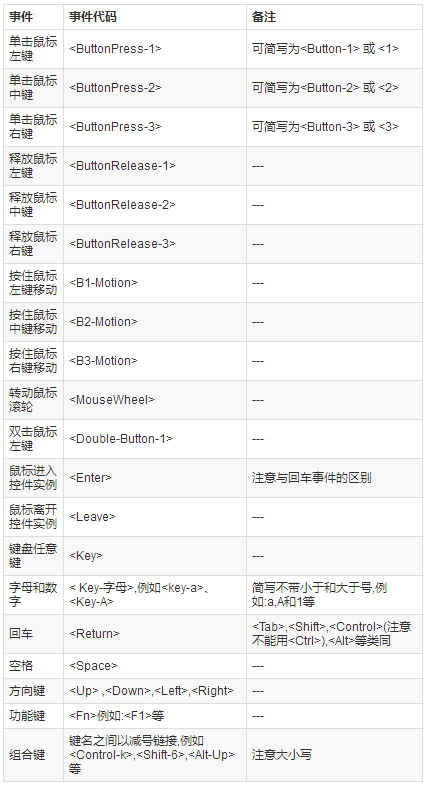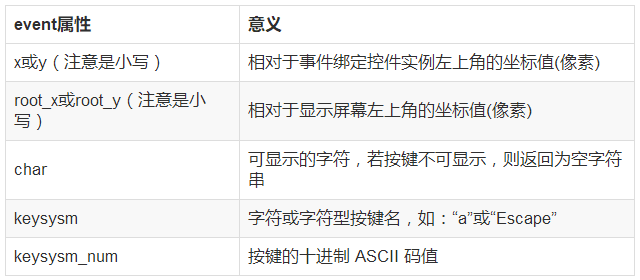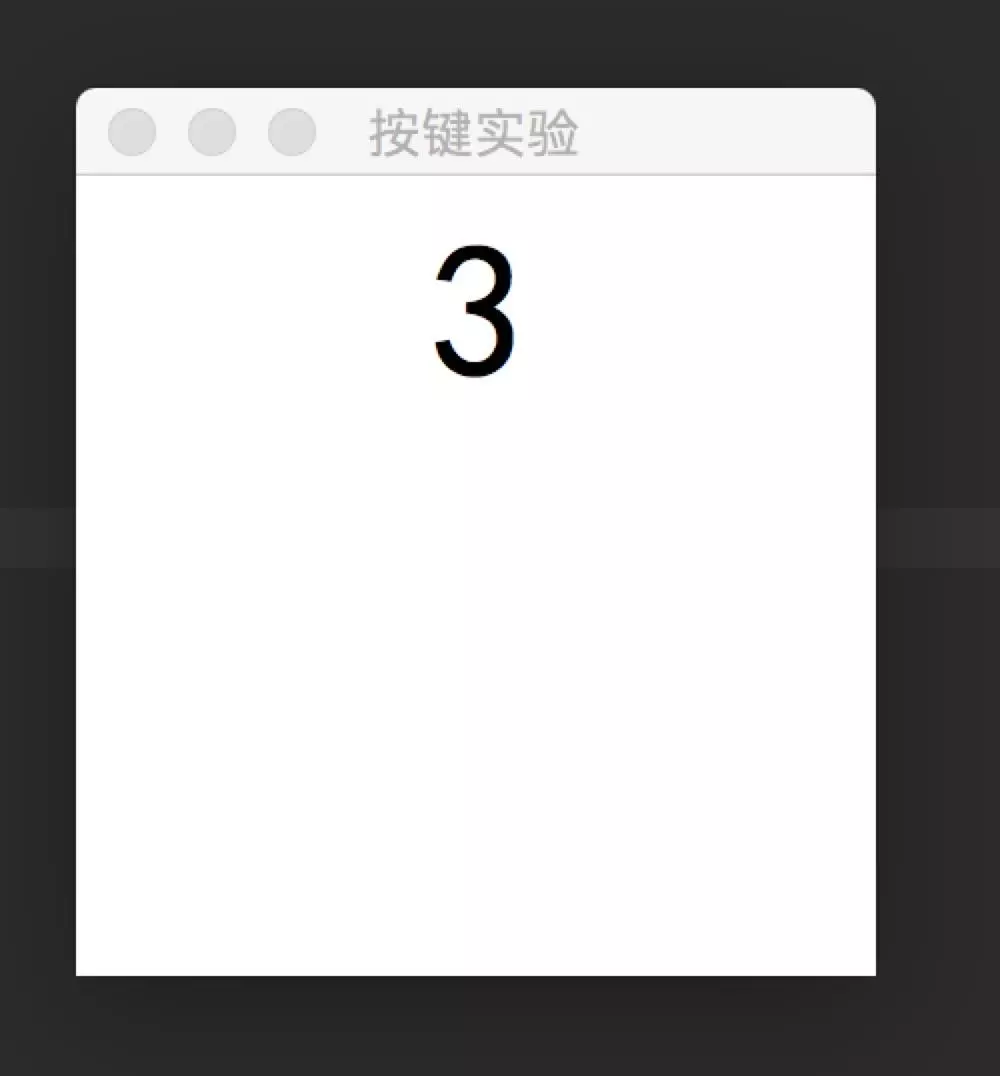from tkinter import *

def show(event):
s=event.keysym
lb.config(text=s)

root=Tk()
root.title('按键实验')
root.geometry('200x200')
lb=Label(root,text='请按键',font=('黑体',48))
lb.bind('<Key>',show)
lb.focus_set()
lb.pack()
root.mainloop()


## 五、背景图片

1、添加背景

#### 返回目录

#插入文件图片
import tkinter as tk

root = tk.Tk()

#创建一个标签类, [justify]:对齐方式
textLabel = tk.Label(root,text="你在右边会看到一个图片，\n我在换个行",
justify = tk.LEFT)#左对齐
textLabel.pack(side=tk.LEFT)#自动对齐,side：方位

#创建一个图片管理类
photo = tk.PhotoImage(file="18.png")#file：t图片路径
imgLabel = tk.Label(root,image=photo)#把图片整合到标签类中
imgLabel.pack(side=tk.RIGHT)#自动对齐

tk.mainloop()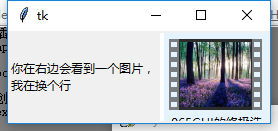#### 返回目录

import tkinter as tk

root = tk.Tk()

#增加背景图片
photo = tk.PhotoImage(file="背景.png")
theLabel = tk.Label(root,
text="我是内容,\n请你阅读",#内容
justify=tk.LEFT,#对齐方式
image=photo,#加入图片
compound = tk.CENTER,#关键:设置为背景图片
font=("华文行楷",20),#字体和字号
fg = "white")#前景色
theLabel.pack()

tk.mainloop()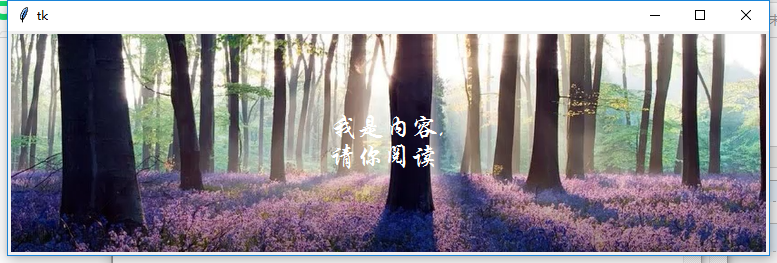#### 返回目录

#插入文件图片
import tkinter as tk

root = tk.Tk()

frame1 = tk.Frame(root)#这是上面的框架
frame2 = tk.Frame(root)#这是下面的框架

var = tk.StringVar()#储存文字的类
var.set("你在右边会看到一个图片，\n我在换个行")#设置文字

#创建一个标签类, [justify]:对齐方式，[frame]所属框架
textLabel = tk.Label(frame1,textvariable=var,
justify = tk.LEFT)#显示文字内容
textLabel.pack(side=tk.LEFT)#自动对齐,side：方位

#创建一个图片管理类
photo = tk.PhotoImage(file="18.png")#file：t图片路径
imgLabel = tk.Label(frame1,image=photo)#把图片整合到标签类中
imgLabel.pack(side=tk.RIGHT)#自动对齐

def callback():#触发的函数
var.set("你还真按了")#设置文字

#[frame]所属框架 ，text 文字内容 command：触发方法
theButton = tk.Button(frame2,text="我是下面的按钮",command=callback)
theButton.pack()#自动对齐

tk.mainloop()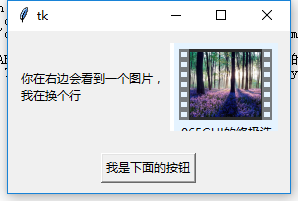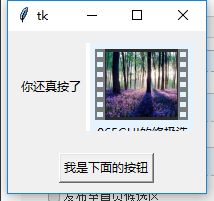## 六、打开摄像头，显示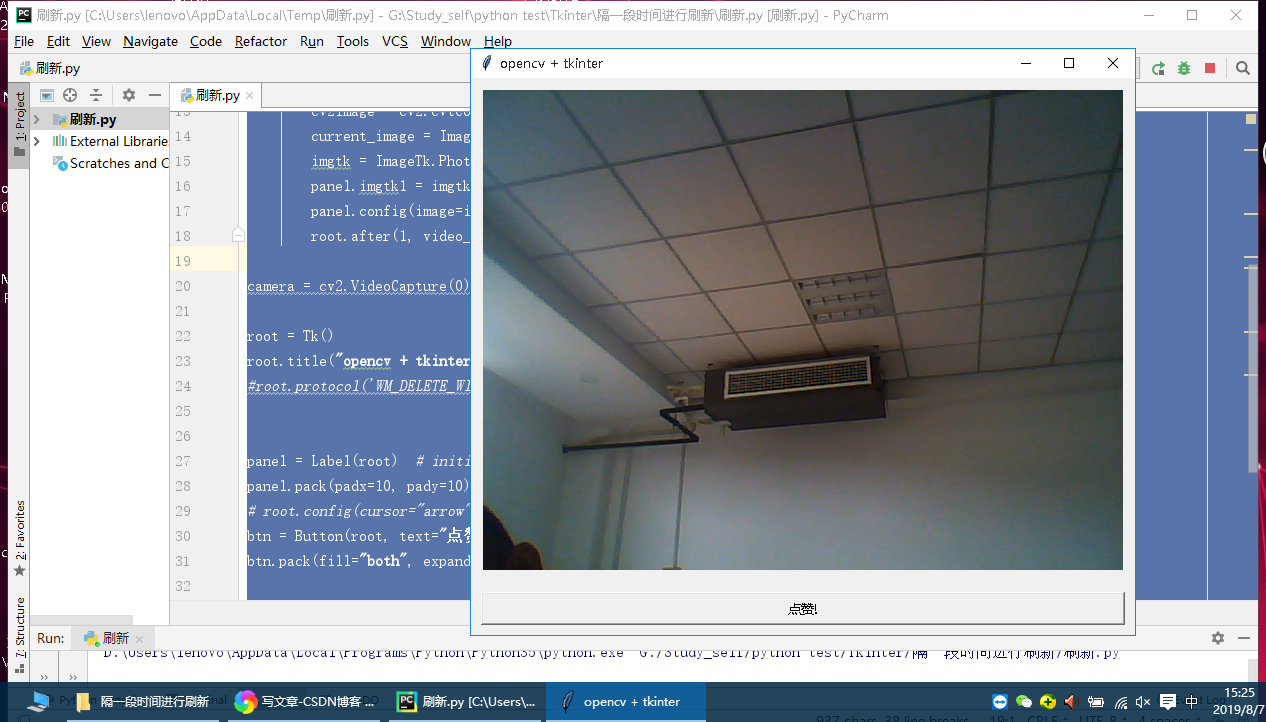from tkinter import *
import cv2
from PIL import Image,ImageTk

def take_snapshot():
print("有人给你点赞啦！")

def video_loop():
success, img = camera.read()  # 从摄像头读取照片
if success:
cv2.waitKey(100)
cv2image = cv2.cvtColor(img, cv2.COLOR_BGR2RGBA)#转换颜色从BGR到RGBA
current_image = Image.fromarray(cv2image)#将图像转换成Image对象
imgtk = ImageTk.PhotoImage(image=current_image)
panel.imgtk1 = imgtk
panel.config(image=imgtk)
root.after(1, video_loop)

camera = cv2.VideoCapture(0)    #摄像头

root = Tk()
root.title("opencv + tkinter")
#root.protocol('WM_DELETE_WINDOW', detector)

panel = Label(root)  # initialize image panel
# root.config(cursor="arrow")
btn = Button(root, text="点赞!", command=take_snapshot)

video_loop()

root.mainloop()
# 当一切都完成后，关闭摄像头并释放所占资源
camera.release()
cv2.destroyAllWindows()


https://www.jianshu.com/p/91844c5bca78
https://www.cnblogs.com/banzhen/p/7427201.html
https://blog.csdn.net/a1_a1_a/article/details/79981788

10-191万+
07-1637608-204017
07-296万+
08-011334
04-271万+
01-262万+
05-241万+
08-221286
09-089万+
04-162万+